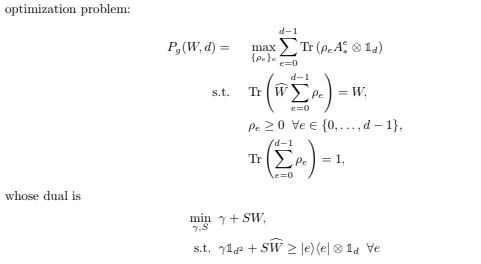# SDP Dual Problem is Giving a Wrong Result

Dear All,
Note: I could not upload the all images since I am a new user so I am adding the link which you can access the the images which are including other information

My optimization problem is that:I am tryin to write code for dual part. But I could not wirte. I mean the program is running but the result is wrong. Probably I could not write the S part correctly Could you please tell me where I am wrong
Best

``````function [G,W,S] = TD_P_inv_Dual(P_exp,T,A_ax,B_by)

d = size(P_exp,1);

m = size(P_exp,3);
%d = size(B_by,1);

%m = size(B_by,3);

cvx_begin

variable S(d,d,m,m)

%variable S2(1,m,m)

W_hat=zeros(d^2,d^2);

for a=1:d

for b=1:d

for x=1:m

for y=1:m

W_hat=W_hat+T(a,b,x,y).*(kron(A_ax(:,:,x,a),B_by(:,:,y,b)));

% if a ~= b

% S=sum(B(a,b,x,y));

% end

%W =W+S(1,x,y)* trace(sigma_e(:,:,a)*kron(A_ax(:,:,2,a),B_by(:,:,2,a)));

% W = trace(kron(A_ax(:,:,2,a),B_by(:,:,2,a)).*sigma_e(:,:,a))

end

end

end

end

W=sum(sum(sum(sum(T.*P_exp))));

J=cvx(0);

for a=1:d

for b =1:d

for x=1:m

for y =1:m

if a ~= b

S(a,b,x,y) == sum(T(a,b,x,y));

%W2=sum(W*S(a,b,x,y));

%disp(W2);

J=S(a,b,x,y).*W;

% W=sum(W*S(a,b,x,y));

%W=W*S(a,b,x,y)

end

end

end

end

end

% for x=1:m

% for y =1:m

%

% J=W*sum(S2(1,x,y));

% %J=W*S2(1,x,y);

% end

% end

%for e=1:d

% W = real(trace(W*rho));

% W = W*trace(sigma_e(:,:,3));

% W = trace(sigma_e(:,:,e)*W);

%end

%J= eye(d)+S*W;

J=real(J)

minimize(J)

subject to

for e=1:d

%(J-kron(squeeze(A_ax(:,:,1,e)),eye(d)))==semidefinite(d^2,d^2);

(J-kron(squeeze(A_ax(:,:,1,e)),eye(d)))==semidefinite(d^2,d^2);

end

%trace(squeeze(sum(S))*W_hat)==W;

cvx_end

S=double(S);

%S=cell2mat(S);

G=double(S(a,b,x,y).*W);

%G2=double(W*rho);``````

my inputs are here:
https://ibb.co/M6NwS7K
https://ibb.co/gwbgnXc

Since I am new user, I could not give link more than 2
https://ibb.co/B3YKJst
https://ibb.co/7rdtgHB

And this for T
https://ibb.co/pnK88ht
https://ibb.co/St8hvCj